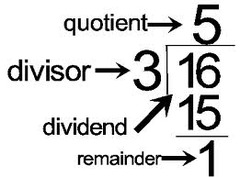Problems
102 - Divisor

Divisor

Time Limit: 1 sec

The Problem

In a division problem, the dividend is D, the quotient is and the remainder is R. What is the divisor?

Example:

if D = 16, Q = 5 and R = 1 then

Divisor is 3The Input

The input file contains only 3 integer numbers D, Q and R (D<=4000, Q<100 and R<20).

The Output

Output will show the one integer numbers in a separate line.

Sample Input

16 5 1

Sample Output

3

Problem Setter: Shahin ShamS